# Chapter 7 Miscellaneous

## 7.1 NCA software

The NCA software is a free package in R called NCA. The first version of the NCA software was released in 2015 just before the online publication of NCA’s core paper on 15 July, 2015. Since then the software was updated several times to correct bugs, integrate new NCA developments, and make improvements based on feedback from users. The following versions have been released so far:

NCA_1.0 (2015-07-02)

NCA_1.1 (2015-10-10)

NCA_2.0 (2016-05-18)

NCA_3.0 (2018-08-01)

NCA_3.0.1 (2018-08-21)

NCA_3.0.2 (2019-11-22)

NCA_3.0.3 (2020-06-11)

NCA_3.1.0 (2021-03-02)

NCA_3.1.1 (2021-05-03)

NCA_3.2.0 (2022-04-05)

When the first digit of the version number increases, major changes were implemented. Version 1 was the first limited version, version 2 was the first comprehensive version, and version 3 includes the statistical test for NCA. The second digit refers to larger changes and the third digit minor changes.

The currently installed version of the NCA software and the availability of a new version can be checked as follows:

packageVersion("NCA") # installed version
available.packages()["NCA",2] # available version

The cumulative number of downloads of the NCA software from the CRAN website are shown in Figure 7.1.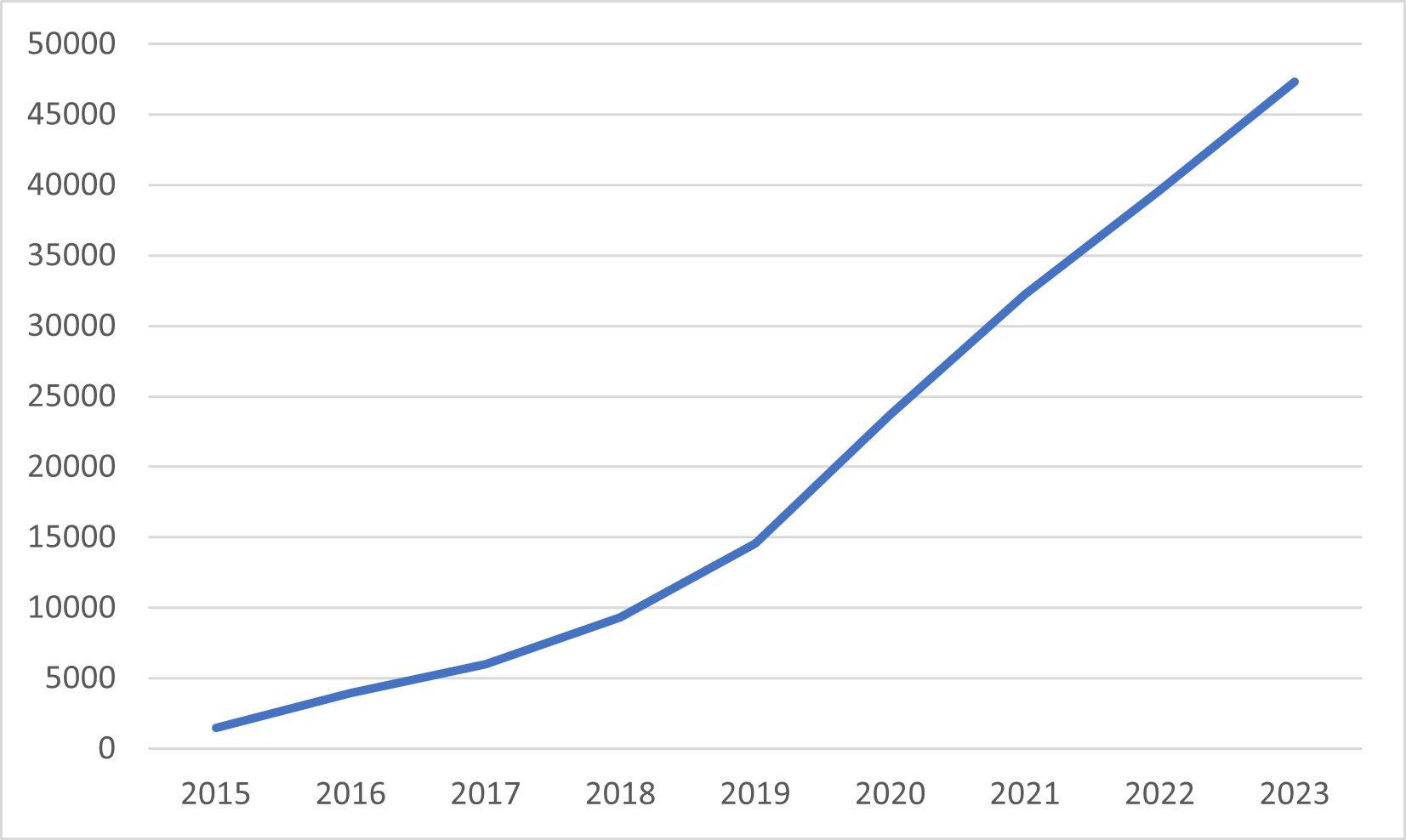Figure 7.1: Cumulative number of downloads from the CRAN website of the NCA software since its launch).

Until 31 December 2021 the software was downloaded more than 30,000 times.

library("cranlogs")
cat("total downloads on")
## total downloads on
Sys.Date()
##  "2022-08-06"
tail(cumsum(x[,2]),n=1)
##  36529

The Quick Start Guide helps novice users of R and NCA to get started, but the guide also includes more advanced features. The NCA package has a build-in help function with examples. Within R, the help function can be called as follows:

help(package = "NCA")

When help is needed for a specific function in NCA, for example nca_analysis the help for this function can be called as follows:

help("nca_analysis")

Currently, the NCA software is not available in statistical packages like SPSS or Stata.

## 7.2 Producing high-quality NCA scatter plots

One of the primary results of NCA is the ‘NCA plot,’ which is a scatter plot with ceiling line(s). Users often wish to save a high quality NCA plot for further use, for example for a publication. There are several ways to make and save a high quality NCA plot. In this section six ways are presented that have different levels of user flexibility:

1. Standard plot.

2. Standard plot with basic adaptations.

6. From within R script.

### 7.2.1 Standard plot

When NCA for R is used with RStudio, the standard NCA plot is displayed in the Plots window of RStudio. The plot can be produced with the argument plots = TRUE of the nca_output function. For the example dataset in NCA package, the standard plot can be obtained as follows:

library (NCA)
data(nca.example)
model <- nca_analysis(nca.example, 1, 3)
nca_output(model, plots = TRUE, summaries = FALSE)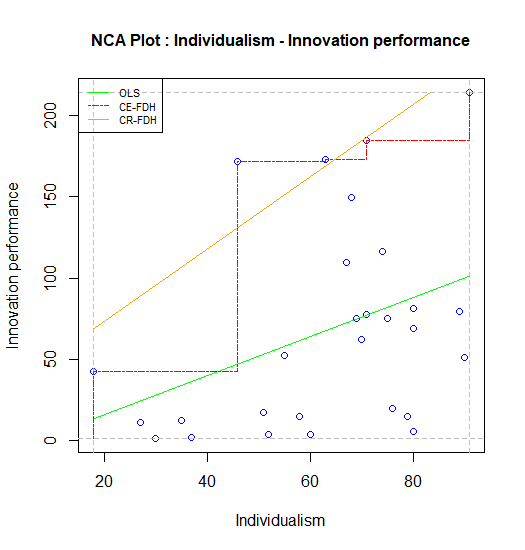Figure 7.2: Standard scatter plot of nca.example.

### 7.2.2 Standard plot with basic adaptations

It may be desirable to change the appearance of the plot, such as the color of the points and the lines, the type of lines and the type of points. These characteristics can be changed with the graphical functions that are included in the NCA software. Before running nca_output, the NCA plot can be customized by changing the point type (for all points), the line types and line colors (for each ceiling line separately) and the line width (for all ceiling lines). For instance, a publisher may require a black and white or grayscale plot, with thicker lines and with solid points. Then the standard plot can be adapted as follows (changing of plot dimensions and the saving the plot is the same as for the standard plot):

line.colors['ce_fdh'] <- 'black'
line.colors['cr_fdh'] <- 'black'
line.colors['ols'] <- 'grey'
point.color <- "black"
point.type <- 20
line.width <- 2
nca_output(model, plots = TRUE, summaries = FALSE)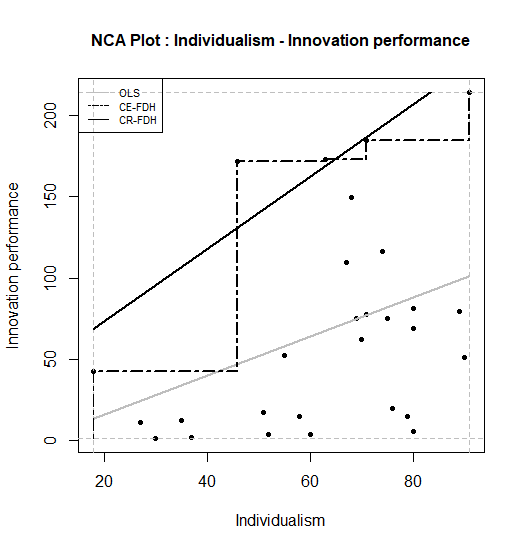Figure 7.3: Standard scatter plot of nca.example in black/grey/white.

If further changes in the plot are needed, for example changes of the individual line types or the plot title, a special R script must be downloaded from: https://stash.ict.eur.nl/projects/NCA/repos/public/browse/display_plot.R?raw This script is used as an alternative for making the NCA plot with plots = TRUE in the nca_output function. The script can be copy/paste or otherwise loaded in your R session.

# Default values copied from p_constants
line_colors <- list(ols="green",        lh="red3",            cols="darkgreen",
qr="blue",          ce_vrs="orchid4",     cr_vrs="violet",
ce_fdh="red",       cr_fdh="orange",      sfa="darkgoldenrod",
c_lp="blue")
line_types  <- list(ols=3,              lh=2,                 cols=3,
qr=4,               ce_vrs=5,             cr_vrs=1,
ce_fdh=6,           cr_fdh=1,             sfa=7,
c_lp=2)
line_width  <- 1.5
point_type  <- 23
point_color <- 'red'
display_plot <-
function (plot) {
flip.x <- plot$flip.x flip.y <- plot$flip.y
# Determine the bounds of the plot based on the scope
xlim <- c(plot$scope.theo[1 + flip.x], plot$scope.theo[2 - flip.x])
ylim <- c(plot$scope.theo[3 + flip.y], plot$scope.theo[4 - flip.y])
# Reset/append colors etc. if needed
for (method in names(line_types)) {
line.types[[method]] <- line_types[[method]]
}
if (is.numeric(line_width)) {
line.width <- line_width
}
if (is.numeric(point_type)) {
point.type <- point_type
}
if (point_color %in% colors()) {
point.color <- point_color
}
# Only needed until the next release (3.0.2)
if (!exists("point.color")) {
point.color <- "blue"
}
# Plot the data points
plot (plot$x, plot$y, pch=point.type, col=point.color, bg=point.color,
xlim=xlim, ylim=ylim, xlab=colnames(plot$x), ylab=tail(plot$names, n=1))
# Plot the scope outline
abline(v=plot$scope.theo, lty=2, col="grey") abline(v=plot$scope.theo, lty=2, col="grey")
abline(h=plot$scope.theo, lty=2, col="grey") abline(h=plot$scope.theo, lty=2, col="grey")
# Plot the legend before adding the clipping area
legendParams = list()
for (method in plot$methods) { line.color <- line.colors[[method]] line.type <- line.types[[method]] name <- gsub("_", "-", toupper(method)) legendParams$names  = append(legendParams$names, name) legendParams$types  = append(legendParams$types, line.type) legendParams$colors = append(legendParams$colors, line.color) } if (length(legendParams) > 0) { legend("topleft", cex=0.7, legendParams$names,
lty=legendParams$types, col=legendParams$colors, bg=NA)
}
# Apply clipping to the lines
clip(xlim, xlim, ylim, ylim)
# Plot the lines
for (method in plot$methods) { line <- plot$lines[[method]]
line.color <- line.colors[[method]]
line.type  <- line.types[[method]]
if (method %in% c("lh", "ce_vrs", "ce_fdh")) {
lines(line[], line[], type="l",
lty=line.type, col=line.color, lwd=line.width)
} else {
abline(line, lty=line.type, col=line.color, lwd=line.width)
}
}
# Plot the title
title(paste0("Advanced Standard NCA Plot : ", plot$title), cex.main=1) } The different default values of the plot can be changed as desired. For example, a researcher may wish to change the ols line into a dotted line (line.type to value 3), and to have a point type that is squared (point.type = 23) with red color of the points (point.color = ‘red’). Also, the title of the plot can be changed at the end of the script into for example “Advanced NCA plot ….” For producing the plot the final call is : display_plot(model$plots[])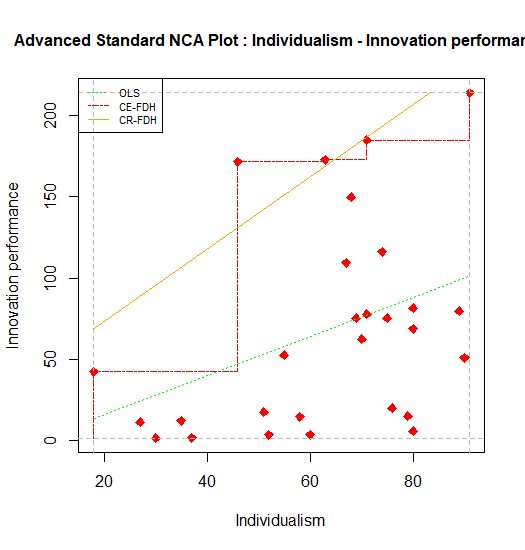The plot can be reset to default values by:

line.colors <- NULL
line.types <- NULL
line.width <- NULL
point.color <- "blue"
point.type <- 21

### 7.2.4 Plot with plotly

The NCA software can also produce a plot made by plotly. The main goal is to provide the user a possibility for graphical exploration of NCA results. However, it is also possible to save the plot. The plot with plotly can be produced with the nca_output function as follows:.

nca_output(model, plotly=TRUE, summaries = FALSE)

It is possible to label subgroups of points. For example, the continents of the countries in the nca.example dataset can be identified as follows (in the order of the rows in the dataset):

labels <- c("Australia", "Europe", "Europe", "North America", "Europe", "Europe", "Europe", "Europe", "Europe", "Europe", "Europe", "Europe", "Europe", "Asia", "North America", "Europe", "Australia", "Europe", "Europe", "Europe", "Europe", "Asia", "Europe", "Europe", "Europe", "Europe", "Europe", "North America")
nca_output(model, plotly=labels, summaries = FALSE)

The plot opens in the Viewer tab of the plots window ((fig:plotly1)). The interactive version can be approached here. The plot identifies the ‘peers’ in red. Peers are points near the ceiling line that are used for drawing the default and other ceiling lines. When moving in the computer screen the pointer over the top of the plot, a toolbar pops up. One of its functions is “Download plot as png” which saves the plot in the download folder of the computer (not in the working directory). Saving is also possible with Export tab -> Save as Image.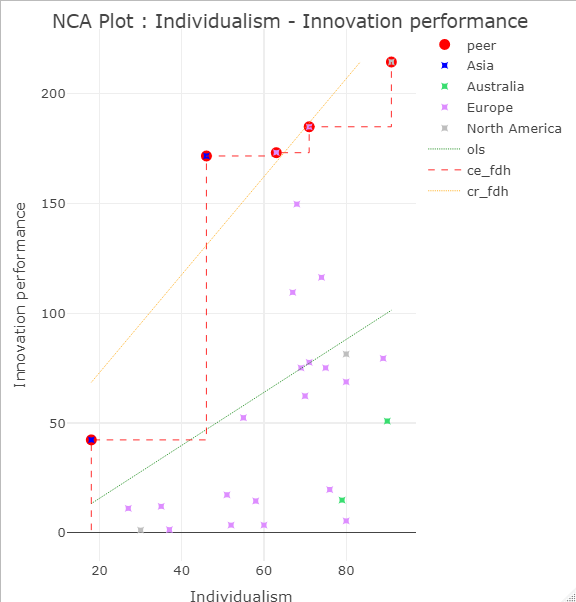Figure 7.5: Plotly scatter plot of nca.example. The interactive version can be approached here.

### 7.2.5 Plot with ggplot

The function ggplot is an advanced graphical function in R. Output of NCA can be used as input for ggplot. For example, when the ceiling line is a straight line (such as the default ceiling line cr_fdh) the intercept and slope of the ceiling line can be used in ggplot to plot the ceiling line. First, these NCA parameters must be extracted from the NCA results

intercept <- model$plots$Individualism$lines$cr_fdh$coefficients slope <- model$plots$Individualism$lines$cr_fdh$coefficients

Next the ggplot can be produced:

library (ggplot2)
ggplot(nca.example, aes(Individualism,Innovation performance))+
geom_point() +
geom_abline(intercept=intercept, slope=slope, col = "red")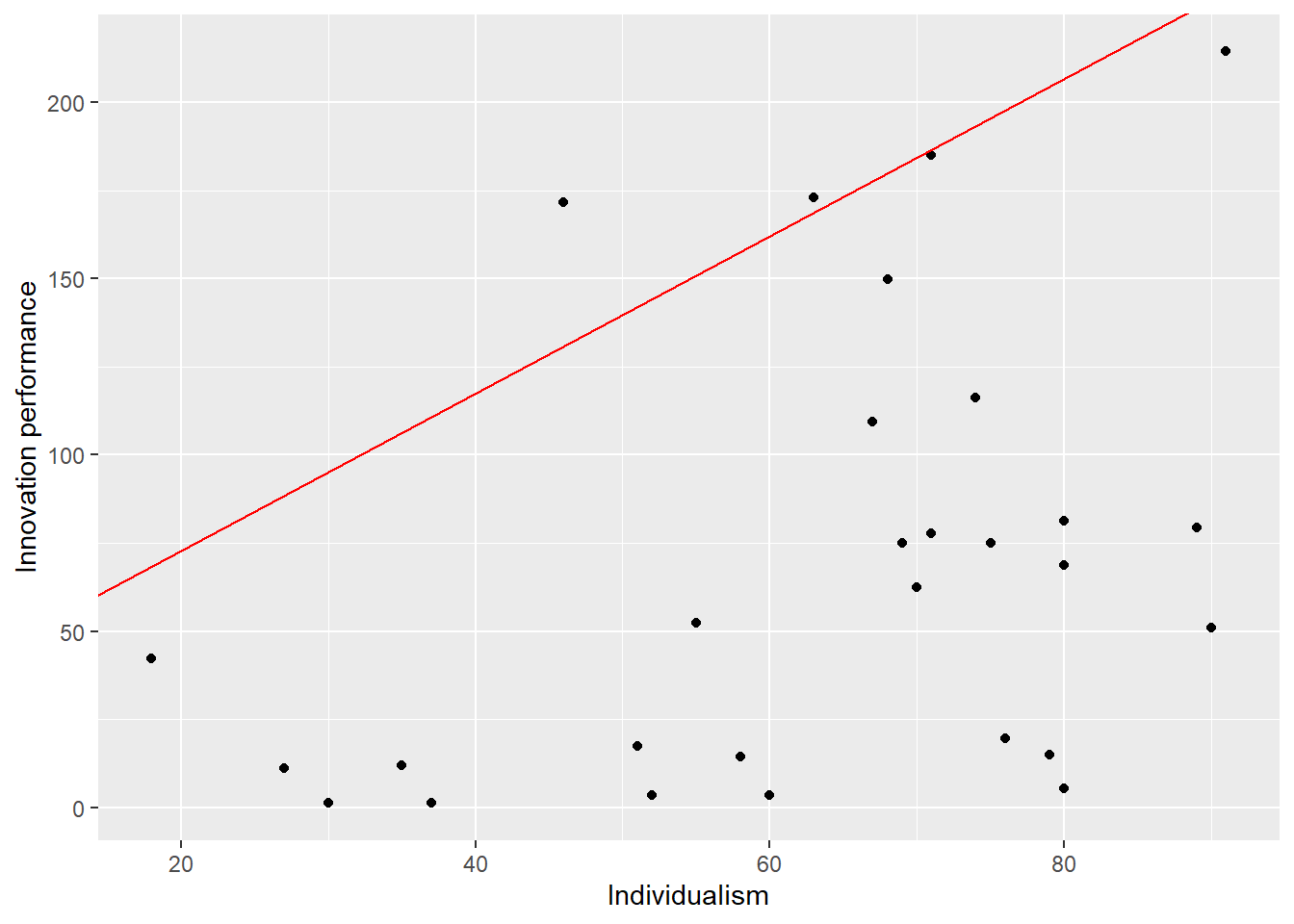Figure 7.6: ggplot scatter plot of nca.example.

The ggplot function gives many graphical opportunities (see its documentation) to make the plot as desired.
Changing of plot dimensions and the saving the plot is the same as for the standard plot.

### 7.2.6 Saving the plot from within the R script

All above options for saving an NCA plot are based on first displaying the plot in R and then saving it. It is also possible to save the plot from within the R script. The following script saves a standard NCA plot as a png file with the name “nca.example.png”) in the working directory with a given size and resolution:

png("nca_example.png",units="cm", 15,15, res=300)
nca_output(model, plots = TRUE, summaries = FALSE)
dev.off() 

It is also possible to save a pdf file:

pdf("nca_example.pdf",5,5)
nca_output(model, plots = TRUE, summaries = FALSE)
dev.off() 

### References

Dul, J. (2016b). Necessary Condition Analysis (NCA) logic and methodology of “necessary but not sufficient” causality. Organizational Research Methods, 19(1), 10–52. https://doi.org/10.1177/1094428115584005